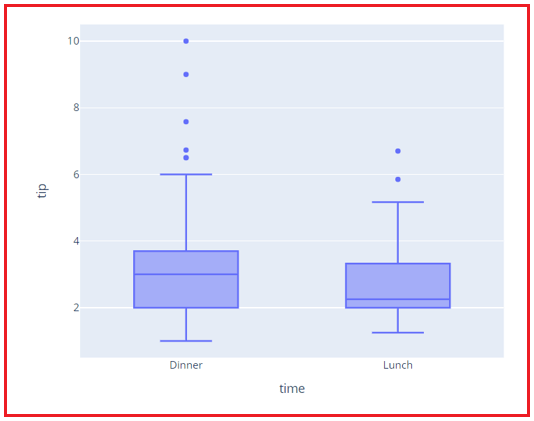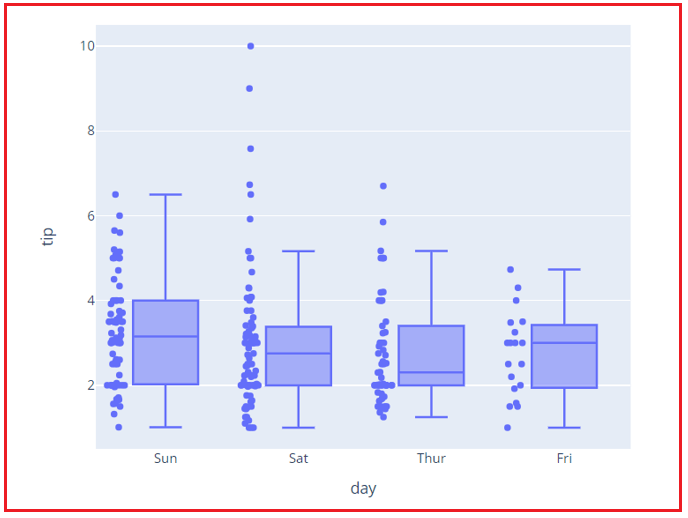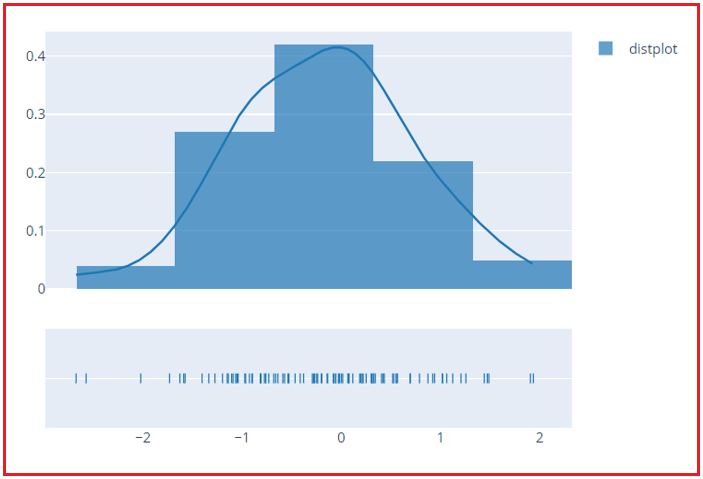# Box and Dist Plots in Python using Plotly

## Box and Dist Plots in Python using Plotly

In this article, I am going to discuss Box and Dist Plots in Python using Plotly for Data Science with Examples. Please read our previous article where we discussed Bar Charts in Python using Plotly with Examples.

##### Box Plots in Python using Plotly

A box plot is a quartile-based demographic depiction of numerical data. The median (second quartile) is indicated by a line inside the box, while the upper and lower quartiles are represented by the edges of the box. All the points that lie outside the box plot are known as outliers, as they represent points farther from the usual data points in the distribution. To create a box plot using plotly, you can use – px.box()

###### Example –

Creating a box plot for a tips dataset to check the distribution of tips for lunch and dinner separately.

```# importing libraries
import plotly.express as px

data = px.data.tips()

# Creating box plot for tips dataset
figure = px.box(data_frame = data, x="time", y="tip")
figure.show()
```
###### Output:From the above plot, we can draw the following conclusions –

1. For Lunch – Tips usually range from 2 to 3.5, while there are tips around 7 approximately as well.
2. For Dinner – Tips usually range from 2 to 3.75, while there are also tips around 10 approximately.

Let’s have a look at this example to check the range of tips on different days. In case you want to display all the underlying points of a column/feature along with the boxplot, you need to use the parameter – points and pass “all” as the value in it.

```# importing libraries
import plotly.express as px

data = px.data.tips()

# Creating box plot for tips dataset
figure = px.box(data_frame = data, x="day", y="tip", points="all")
figure.show()
```
###### Output:From the above plot, we can draw the following conclusions –

1. The range of tips is higher on Sundays.
2. Maximum tips can be seen on Saturdays
3. Comparatively, on Thursday and Friday, we can see less range of tips.
##### Dist Plots in Python using Plotly

A distribution plot, also known as a Distplot, displays the variation in data distribution. The total distribution of continuous data variables is shown by the Seaborn Distplot. The data is represented by a combination of the two of these – rug plot, histogram, and a line in the Distplot. The create_distplot() function in the figure_factory module requires a necessary parameter called hist data.

###### Example –
```# importing libraries
import numpy as np
import plotly.figure_factory as ff

hist_data = [np.random.randn(100)]
labels = ['distplot']
figure = ff.create_distplot(hist_data, labels)
figure.show()
```
###### Output:In the next article, I am going to discuss Histograms and Heatmaps in Python using Plotly for Data Science with Examples. Here, in this article, I try to explain Box Plots and Dist Plots in Python using Plotly for Data Science with Examples. I hope you enjoy this Box Plots and Dist Plots in Python using Plotly for Data Science article.